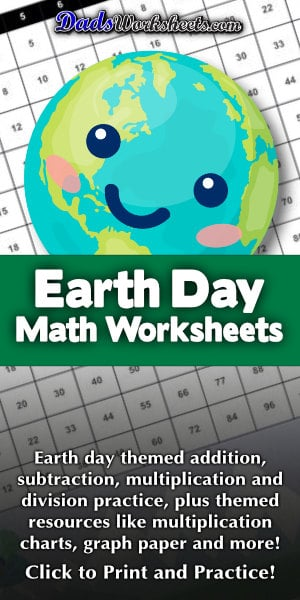One Dad. Four daughters. 9,393 worksheets... and counting!

# Earth Day

Looking for worksheets to make learning math on Earth Day a bit more fun? This page has a collection of color by number worksheets appropriate for kindergarten through fourth grade, covering addition, subtraction, multiplication and division operations. There are also a collection of simple math exercises with fun Earth Day themes.

## Celebrate Earth Day with Some Math Practice!

If you're celebrating Earth Day, it can be a lot of fun to introduce some math practice to go along with your explorations of recycling, conservation and climate science!

The worksheets on this page feature both math fact practice and printable paper for homework, test taking and other activities. You'll find Earth day themed math worksheets covering addition, subtraction, multiplication and division.

These worksheets are appropriate for students in kindergarten through 4th grade. The addition and subtraction worksheets are appropriate for first grade and second grade students, while the multiplication and division worksheets more appropriate for third and 4th grade students.